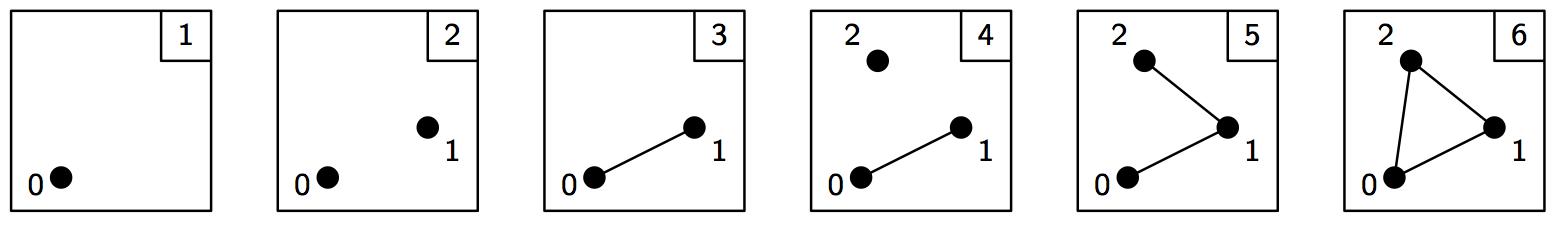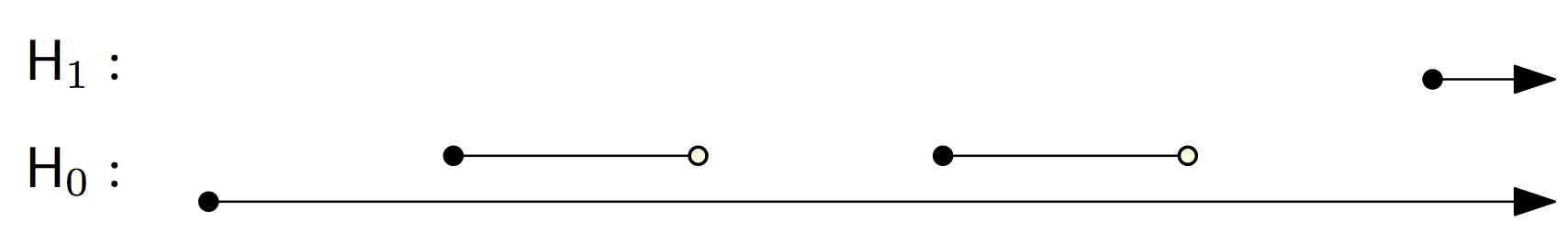# Basics¶

First, we import everything from Dionysus:

>>> from __future__ import print_function   # if you are using Python 2
>>> import dionysus as d


## Simplices¶

A simplex is simply a list of vertices. It’s represented by the Simplex class:

>>> s = d.Simplex([0,1,2])
>>> print("Dimension:", s.dimension())
Dimension: 2


We can iterate over the vertices of the simplex.

>>> for v in s:
...     print(v)
0
1
2


Or over the boundary:

>>> for sb in s.boundary():
...     print(sb)
<1,2> 0
<0,2> 0
<0,1> 0


Simplices can store optional data, and the 0 reported after each boundary edge is the default value of the data:

>>> s.data = 5
>>> print(s)
<0,1,2> 5


We can use closure() to generate all faces of a set of simplices. For example, an 8-sphere is the 8-dimensional skeleton of the closure of the 9-simplex.

>>> simplex9 = d.Simplex([0,1,2,3,4,5,6,7,8,9])
>>> sphere8  = d.closure([simplex9], 8)
>>> print(len(sphere8))
1022


## Filtration¶

A filtration is a nested sequence of simplicial complexes, $$K_1 \subseteq K_2 \subseteq \ldots \subseteq K_n$$. Without loss of generality, we can assume that two consecutive complexes in the filtration differ by a single simplex, so we can think of a filtration as a sequence of simplices.In Dionysus, a filtration is represented by a special class, Filtration, that supports both iterating over the simplices and looking up an index given a simplex. A filtration can be sort()ed. By default this orders simplices by their data, breaking ties by dimension, and then lexicographically.

>>> simplices = [(, 4), ([1,2], 5), ([0,2], 6),
...              (, 1),   (, 2), ([0,1], 3)]
>>> f = d.Filtration()
>>> for vertices, time in simplices:
...     f.append(d.Simplex(vertices, time))
>>> f.sort()
>>> for s in f:
...    print(s)
<0> 1
<1> 2
<0,1> 3
<2> 4
<1,2> 5
<0,2> 6


We can lookup the index of a given simplex. (Indexing starts from 0.)

>>> print(f.index(d.Simplex([1,2])))
4


## Persistent Homology¶

Applying homology functor to the filtration, we get a sequence of homology groups, connected by linear maps: $$H_*(K_1) \to H_*(K_2) \to \ldots \to H_*(K_n)$$. To compute decomposition of this sequence, i.e., persistence barcode, we use homology_persistence(), which returns its internal representation of the reduced boundary matrix:

 >>> m = d.homology_persistence(f)
>>> for i,c in enumerate(m):
...     print(i, c)
0
1
2 1*0 + 1*1
3
4 1*1 + 1*3
5We can manually extract the persistence pairing from the reduced matrix:

>>> for i in range(len(m)):
...     if m.pair(i) < i: continue      # skip negative simplices
...     dim = f[i].dimension()
...     if m.pair(i) != m.unpaired:
...         print(dim, i, m.pair(i))
...     else:
...         print(dim, i)
0 0
0 1 2
0 3 4
1 5


But we can also use the init_diagrams() function, by providing it access to the filtration:

>>> dgms = d.init_diagrams(m, f)
>>> print(dgms)
[Diagram with 3 points, Diagram with 1 points]
>>> for i, dgm in enumerate(dgms):
...     for pt in dgm:
...         print(i, pt.birth, pt.death)
0 1.0 inf
0 2.0 3.0
0 4.0 5.0
1 6.0 inf


Notice that init_diagrams() uses the data stored in the simplices, instead of the index pairing we printed out in the previous example.

homology_persistence() knows several methods of persistence computation. These can be specified with the method keyword argument:

>>> m = d.homology_persistence(f, method = 'column')


Homology. Dionysus doesn’t compute homology directly, but we can get it as a by-product of persistent homology.

>>> f = d.Filtration(sphere8)
>>> f.sort()
>>> m = d.homology_persistence(f, prime=2)
>>> dgms = d.init_diagrams(m, f)
>>> for i, dgm in enumerate(dgms):
...     print("Dimension:", i)
...     for p in dgm:
...         print(p)
Dimension: 0
(0,inf)
Dimension: 1
Dimension: 2
Dimension: 3
Dimension: 4
Dimension: 5
Dimension: 6
Dimension: 7
Dimension: 8
(0,inf)


### Relative persistent homology¶

It’s possible to compute persistent homology of a filtration relative to a subcomplex: $$H_*(K_1, L_1) \to H_*(K_2, L_2) \to \ldots \to H_*(K_n, L_n)$$, where $$L_i = K_i \cap L_n$$. To accomplish this, homology_persistence() takes an extra argument, relative, to specify the subcomplex, $$L_n$$. This subcomplex is represented by a Filtration, but the ordering of the simplices in it doesn’t matter, only their presence. For example, homology of a triangle relative to its boundary has a single class in dimension 2:

>>> f = d.Filtration(d.closure([d.Simplex([0,1,2])], 2))
>>> f.sort()
>>> f1 = d.Filtration([s for s in f if s.dimension() <= 1])

>>> m = d.homology_persistence(f, relative = f1)
>>> dgms = d.init_diagrams(m, f)
>>> for i, dgm in enumerate(dgms):
...     print("Dimension:", i)
...     for p in dgm:
...         print(p)
Dimension: 0
Dimension: 1
Dimension: 2
(0,inf)


## Diagram Distances¶

wasserstein_distance() computes q-th Wasserstein distance between a pair of persistence diagrams. bottleneck_distance() computes the bottleneck distance.

>>> f1 = d.fill_rips(np.random.random((20, 2)), 2, 1)
>>> m1 = d.homology_persistence(f1)
>>> dgms1 = d.init_diagrams(m1, f1)
>>> f2 = d.fill_rips(np.random.random((20, 2)), 2, 1)
>>> m2 = d.homology_persistence(f2)
>>> dgms2 = d.init_diagrams(m2, f2)
>>> wdist = d.wasserstein_distance(dgms1, dgms2, q=2)
>>> print("2-Wasserstein distance between 1-dimensional persistence diagrams:", wdist)
2-Wasserstein distance between 1-dimensional persistence diagrams: 0.06525366008281708
>>> bdist = d.bottleneck_distance(dgms1, dgms2)
>>> print("Bottleneck distance between 1-dimensional persistence diagrams:", bdist)
Bottleneck distance between 1-dimensional persistence diagrams: 0.060736045241355896


## Homologous Cycles¶

To determine if two chains are homologous, use homologous() method:

>>> simplices = [, , [0,1], ]
>>> f = d.Filtration(simplices)
>>> f.sort()
>>> m = d.homology_persistence(f)

>>> m.homologous(d.Chain([(1,0)]), d.Chain([(1,1)]))
True

>>> m.homologous(d.Chain([(1,0)]), d.Chain([(1,2)]))
False